Next: Term-document matrices and singular Up: Linear algebra review Previous: Linear algebra review   Contents   Index

## Matrix decompositions

In this section we examine ways in which a square matrix can be factored into the product of matrices derived from its eigenvectors; we refer to this process as matrix decomposition . Matrix decompositions similar to the ones in this section will form the basis of our principal text-analysis technique in Section 18.3 , where we will look at decompositions of non-square term-document matrices. The square decompositions in this section are simpler and can be treated with sufficient mathematical rigor to help the reader understand how such decompositions work. The detailed mathematical derivation of the more complex decompositions in Section 18.2 are beyond the scope of this book.

We begin by giving two theorems on the decomposition of a square matrix into the product of three matrices of a special form. The first of these, Theorem 18.1.1, gives the basic factorization of a square real-valued matrix into three factors. The second, Theorem 18.1.1, applies to square symmetric matrices and is the basis of the singular value decomposition described in Theorem 18.2.

Theorem. (Matrix diagonalization theorem) Let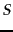be a square real-valued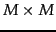matrix with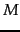linearly independent eigenvectors. Then there exists an eigen decomposition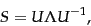(223)

where the columns of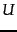are the eigenvectors ofand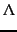is a diagonal matrix whose diagonal entries are the eigenvalues ofin decreasing order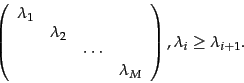(224)

If the eigenvalues are distinct, then this decomposition is unique. End theorem.

To understand how Theorem 18.1.1 works, we note thathas the eigenvectors ofas columns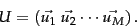(225)

Then we have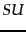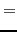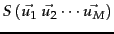(226)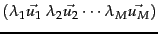(227)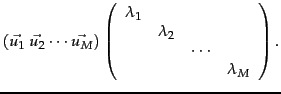(228)

Thus, we have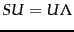, or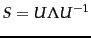.

We next state a closely related decomposition of a symmetric square matrix into the product of matrices derived from its eigenvectors. This will pave the way for the development of our main tool for text analysis, the singular value decomposition (Section 18.2 ).

Theorem. (Symmetric diagonalization theorem) Letbe a square, symmetric real-valuedmatrix withlinearly independent eigenvectors. Then there exists a symmetric diagonal decomposition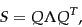(229)

where the columns of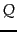are the orthogonal and normalized (unit length, real) eigenvectors of, andis the diagonal matrix whose entries are the eigenvalues of. Further, all entries ofare real and we have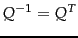. End theorem.

We will build on this symmetric diagonal decomposition to build low-rank approximations to term-document matrices.

Exercises.

• What is the rank of the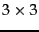diagonal matrix below?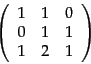(230)

• Show that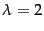is an eigenvalue of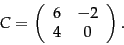(231)

Find the corresponding eigenvector.

• Compute the unique eigen decomposition of the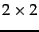matrix in (222).Next: Term-document matrices and singular Up: Linear algebra review Previous: Linear algebra review   Contents   Index